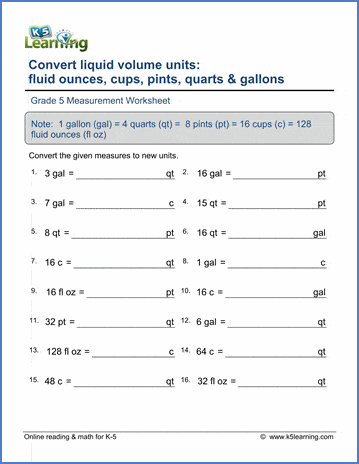## Measurement worksheets

Our grade 5 measurement worksheets give students practice in converting between different measurement units for length, mass and volume and in converting units of measurement between the customary and metric systems.

## Lengths

Convert between inches, feet and yards 8 yd  = ___ in
Convert between miles, feet and yards 23 mi = ___ yd

Convert between mm, cm, m and km 857 km = ___ m
Convert between mm, cm, m and km (decimals) 4.033 m = ___ mm

Convert lengths: customary / metric 57 in = ___ mm
Convert longer lengths: customary / metric 84 km = ___ yd

## Weights

Convert between ounces, pounds and tons 25 t = ____ lbs
Convert between metric weights (gm & kg) 9.7 kg = ___ gm
Convert weights between customary and metric 45 lb = ___gm

## Capacities (Volumes)

Convert cups, pints, quarts and gallons  290 qt = ___ gal
Convert ounces, cups, pints, quarts and gallons 8 qt = ___ pt
Convert volumes using decimals 2.5 qt = ___ pt
Convert metric capacities 0.89 l = ___ ml
Convert customary and metric volumes 30 gal = ___ l

## Mixed practice (length, weight, capacity)

Customary units - mixed practice 296 oz = ___ gal
Customary units - harder practice 38 lb = ____ t
Metric units - mixed practice 9.995 kg = ___ g
Mixed customary-metric conversions 46 ft = ___ cm

## Measurement word problems

Length word problems Word problems
Mass / weight word problems Word problems
Volume / capacity word problems Word problems